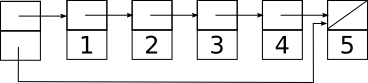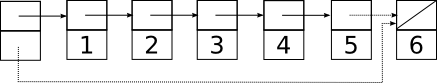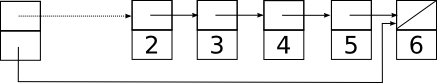# Queues

Stacks are last-in-first-out (LIFO) data structures: when we pop, we get the last item we pushed. What if we want a first-in-first-out (FIFO) data structure? Such a data structure is called a queue and can also be implemented by a linked list. The difference is that if we want O(1) time for both the enqueue (push) and dequeue (pop) operations, we must keep around pointers to both ends of the linked list.

So now we get something that looks like this:Enqueuing a new element typically requires (a) allocating a new struct to hold it; (b) making the old tail struct point at the new struct; and (c) updating the tail pointer to also point to the new struct. There is a minor complication when the stack is empty; in this case instead of updating tail->next we must put a pointer to the new struct in head. Dequeuing an element involves updating the head pointer and freeing the removed struct, exactly like a stack pop.

Here is the queue above after enqueuing a new element 6. The updated pointers are indicated by dotted lines:Because we are only changing two pointers, each of which we can reach by following a constant number of pointers from the main struct, we can do this in O(1) time.

There is a slight complication when we enqueue the very first element, because we need to update the head pointer instead of the pointer in the previous tail (which doesn’t yet exist). This requires testing for an empty queue in the enqueue routine, which we’ll do in the sample code below.

Dequeuing is easier because it requires updating only one pointer:If we adopt the convention that a null in head means an empty queue, and use this property to check if the queue is empty when enqueuing, we don’t even have to clear out tail when we dequeue the last element.

Here is a simple implementation of a queue holding ints, together with some test code showing how its behavior differs from a stack:

#include <stdio.h>
#include <stdlib.h>
#include <assert.h>

/* standard linked list element */
struct elt {
struct elt *next;
int value;
};

struct queue {
struct elt *head;  /* dequeue this next */
struct elt *tail;  /* enqueue after this */
};

/* create a new empty queue */
struct queue *
queueCreate(void)
{
struct queue *q;

q = malloc(sizeof(struct queue));

return q;
}

/* add a new value to back of queue */
void
enq(struct queue *q, int value)
{
struct elt *e;

e = malloc(sizeof(struct elt));
assert(e);

e->value = value;

/* Because I will be the tail, nobody is behind me */
e->next = 0;

/* If the queue was empty, I become the head */
} else {
/* Otherwise I get in line after the old tail */
q->tail->next = e;
}

/* I become the new tail */
q->tail = e;
}

int
queueEmpty(const struct queue *q)
{
}

/* remove and return value from front of queue */
int
deq(struct queue *q)
{
int ret;
struct elt *e;

assert(!queueEmpty(q));

/* patch out first element */

free(e);

return ret;
}

/* print contents of queue on a single line, head first */
void
queuePrint(struct queue *q)
{
struct elt *e;

for(e = q->head; e != 0; e = e->next) {
printf("%d ", e->value);
}

putchar('\n');
}

/* free a queue and all of its elements */
void
queueDestroy(struct queue *q)
{
while(!queueEmpty(q)) {
deq(q);
}

free(q);
}

int
main(int argc, char **argv)
{
int i;
struct queue *q;

q = queueCreate();

for(i = 0; i < 5; i++) {
printf("enq %d\n", i);
enq(q, i);
queuePrint(q);
}

while(!queueEmpty(q)) {
printf("deq gets %d\n", deq(q));
queuePrint(q);
}

queueDestroy(q);

return 0;
}